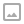## 「SDOI2010」古代猪文-Lucas+CRT

·  ✏️ About  962 words  ·  ☕ 2 mins read · 👀... views

iPig 在大肥猪学校图书馆中查阅资料，得知远古时期猪文文字总个数为 $N$ 。当然，一种语言如果字数很多，字典也相应会很大。当时的猪王国国王考虑到如果修一本字典，规模有可能远远超过康熙字典，花费的猪力、物力将难以估量。故考虑再三没有进行这一项劳猪伤财之举。当然，猪王国的文字后来随着历史变迁逐渐进行了简化，去掉了一些不常用的字。

iPig 打算研究古时某个朝代的猪文文字。根据相关文献记载，那个朝代流传的猪文文字恰好为远古时期的 $k$ 分之一，其中 $k$ 是 $N$ 的一个正约数（可以是 $1$ 和 $N$ ）。不过具体是哪 $k$ 分之一，以及 $k$ 是多少，由于历史过于久远，已经无从考证了。

iPig觉得只要符合文献，每一种能整除 $N$ 的 $k$ 都是有可能的。他打算考虑到所有可能的 $k$ 。显然当 $k$ 等于某个定值时，该朝的猪文文字个数为 $\frac{N}{k}$。然而从 $N$ 个文字中保留下 $\frac{N}{k}$ 个的情况也是相当多的。iPig预计，如果所有可能的 $k$ 的所有情况数加起来为 $P$ 的话，那么他研究古代文字的代价将会是 $G$ 的 $P$ 次方。

## 题解

$$t = \sum_{k|N} C^{k}_{N}\ ans = G^t$$

$$a ^ b \equiv a ^ {b\ \bmod\ p-1} \pmod p$$

$$ans = G^t = G^{t\ \bmod\ (p-1)} \pmod p$$

## 代码

  1 2 3 4 5 6 7 8 9 10 11 12 13 14 15 16 17 18 19 20 21 22 23 24 25 26 27 28 29 30 31 32 33 34 35 36 37 38 39 40 41 42 43 44 45 46 47 48 49 50 51 52 53 54 55 56 57 58 59 60 61 62 63 64 65 66 67 68 69 70  #include #include #include #define ll long long using namespace std; const ll P = 999911659; const int MAXN = 100000; ll pow(ll x,ll k,ll p){ ll ans = 1; for(ll i = k;i;i>>=1,x = x*x%p) if(i & 1) ans = (ans * x) % p; return ans; } ll inv(ll x,ll p){return pow(x,p-2,p);} ll mod = {2,3,4679,35617},pw[MAXN]; void init(ll p){ pw = 1; for(int i = 1;i n) return 0; return (pw[n] * inv(pw[m],p) % p) * inv(pw[n-m],p) % p; } ll lucas(ll n,ll m,ll p){ if(!m) return 1; return c(n%p,m%p,p) * lucas(n/p,m/p,p) % p; } ll N,G,ans; ll CRT(){ ll sum = 0; for(int i = 0;i<4;i++){ ll now = (P-1)/mod[i]; sum = sum + ans[i] * now * inv(now,mod[i]); sum %= (P-1); } return sum; } ll solve(){ for(int num = 0;num<4;num++){ ll p = mod[num],q = sqrt(N); init(p); ans[num] = 0; for(int i = 1;i<=q;i++){ if(N % i != 0) continue; ans[num] = ans[num] + lucas(N,i,p); if(i * i == N) continue; ans[num] = ans[num] + lucas(N,N/i,p); ans[num] %= p; } } return CRT(); } int main(){ scanf("%lld %lld",&N,&G); if(G == P) printf("%d\n",0); else printf("%lld\n",pow(G,solve(),P)); return 0; }WRITTEN BY
cqqqwq
A student in Computer Science.